#### Option 3.2.4. Average

Select Average in the Calculation field on the Virtual tab of the Point Properties dialog box.

An Average point maintains the average value for the source Point ID that you specify in the Expression field. This virtual point can be a signed or unsigned integer, or a floating-point number.

The average is calculated as the accumulation of the deviation from the average point data, divided by the number of samples taken. The calculation is:

average = average + (source - average) / sample_count

or simply as

average + (source - average) / sample_count

The average is calculated as an eight-byte floating-point data type, then the result is converted into the data type you specify for the average point.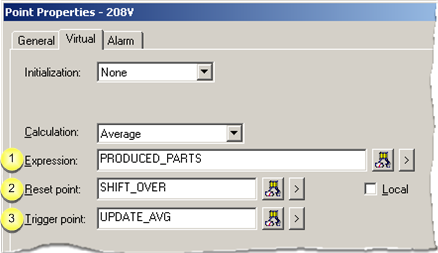Expression Reset point Trigger point

 Expression

Enter an expression that calculates the value of the Average point in the Expression field on the Virtual tab of the Point Properties dialog box.

The expression will be monitored for transitions.

The expression may contain: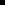One or more Point IDs as well asConstant values, operations, and functions.

Buttons to help with the equation are as follows.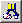Opens the Expression Editor. Displays a Popup menu to: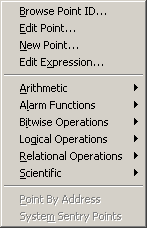Open: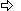The Select a Point browser.The Point Properties dialog box for a selected point.A New Point dialog box.The Expression Editor.Display an extended menu with the associated operations.

 Reset point field

Based on whether there is an entry in the Reset Point field, at runtime the Average point is reset as follows:

 When the trigger point is not defined If And Then An Initial Average Point Value is The Current value of the Expression is The Average point value is reset to the: Not defined Unavailable Zero Not defined Available Current value of the source point Defined Unavailable Initial value Defined Available Average of the source and initialized value When the trigger point is defined If Then An Initial Average Point Value is The Average point value is reset to the: Not defined Unavailable* Defined Initial value*

*    When the trigger point is defined, the result will either be the source value or the average of the source and initialized value.

Note: Buttons to the right of the Reset Point and Trigger Point fields help with configuration.Opens the Select a Point browser to select an available point. Displays a Popup menu to: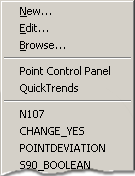Open:A New Point dialog box.The Point Properties dialog box for a selected point.The Select a Point browser.Select a recently selected point.

 Trigger point

When the value of the Average point updates depends on whether or not you defined the trigger point as follows:

 Trigger point The Value of the Average Point is updated When the Value of the: Defined Trigger point is updated. Not defined Source point in the Expression field is updated.

Buttons to help with the trigger point have the same functionality as the buttons to the right of the Reset Point field.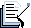Note: For integer type points, the resulting data is rounded. This may result in a loss of accuracy. It is suggested that you use the Real point type for your Average point.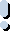Important: Average points are not supported in redundancy.

 Step 3.2. Configure virtual calculations.